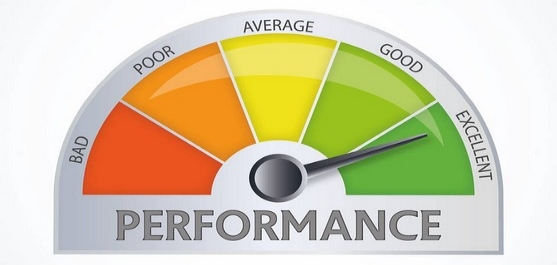## Kaggle evaluation metrics used for regression problems

February 16, 2019This post describe evaluation metrics used in Kaggle competitions where problem to solve is has regression nature. Eight different metrics are described, namely: Absolute Error (AE), Mean Absolute Error (MAE), Weighted Mean Absolute Error (WMAE), Pearson Correlation Coefficient, Spearman’s Rank Correlation, Root Mean Squared Error (RMSE), Root Mean Squared Logarithmic Error (RMSLE), Mean Columnwise Root Mean Squared Error (MCRMSE).

## What’s cooking

April 05, 2018Exploratory Data Analysis of the Kaggle’s “What’s cooking” competition dataset to get understanding what kind of data we are dealing with and get intuition of existing dependencies.